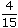# Aptitude - Ratio and Proportion - Discussion

Discussion Forum : Ratio and Proportion - General Questions (Q.No. 1)
1.
A and B together have Rs. 1210. Ifof A's amount is equal toof B's amount, how much amount does B have?
Rs. 460
Rs. 484
Rs. 550
Rs. 664
Explanation:

 4 A = 2 B 15 5A =2 x 15B 5 4A = 3 B 2A = 3 B 2A : B = 3 : 2.B's share = Rs.1210 x 2= Rs. 484. 5

Discussion:
143 comments Page 1 of 15.

I have one more solution:

Here a+b=1210

All given possible values of b in options are :

1. 460 2. 484
3. 550 4. 664

By b's we can find a's values

1. (1210-460)=750 2. (1210-484)=726
3. (1210-550)=660 4. (1210-664)=546

Condition given: 4/15a = 2/5b then
For b's values we will check condition

1. (4/15)*750 = (2/5)*460
200 = 184 (false)

2. (4/15)*726 = (2/5)*484
193.6 = 193.6 (true)

3. (4/15)*660 = (2/5)*550
176 = 220 (false)

4. (4/15)*546 = (2/5)*664
145.6 = 265.6 (false)

So b=484 is correct.

Sandeep said:   7 years ago
Here,

A + B = 12110.
A 4/15 = B 2/5.
It can be written as per the rules of the ratio.

4/15 = 4 :15 and 2/5 = 2:5.

As per rules of ratio:
If a : b = c : d then a : b :: c : d in short it equal to ad : bc.

So, here 4 : 15 = 2 : 5.

Then,
4:15 :: 2:5 = 4 * 5 : 15 * 2 = 20 : 30.

2 : 3 So, the ratio between a and b is 2 : 3.

=> a + b = 1210
So, a : b =2 : 3.
b =1210 * 3/5.
=726.

Am I correct? Is there any mistake please tell me.

Pravallika said:   7 years ago
@Sandeep

The ratio a : b is different from that of ad : bc.
2 : 3 is the answer for ad : bc but not a : b.

A(4/15) = B(2/5).

As per the rules of the ratio, it is written as;
4/15 = 4 :15 and 2/5 = 2:5.

Because they didn't mention the ratios of the amounts they had. They mentioned about the relation between those ratios.

A(4/15) = B(2/5) then A : B is 3 : 2.

And amount of B is 3/(3 + 2) * 1210 = 484.

Sivaa said:   4 years ago
A + B = 1210.

Now find the value of B?
4/15 A = 2/5 B.
A = (2/5 * 15/4) B.
==> A = (3/2) B.
ratio is A : B = 3:2 ==> ratio of A is 3 and ratio of B is 2.
A + B = 1210 // this formula Apply the A value,
(3/2) B + B = 1210 // ratio of B is 2 so B is replace 2.
(3)B + 2 = 1210 * 2,
(3)B + 2 = 2420,
5(B) = 2420,
B = 2420/5,
B = 484.

Shannu said:   5 years ago
Can you give solution for this problem?

A vessel of 125 lts. Capacity is full of pure milk on the first day 2/5 of the milk is drawn and filled with water, on the second day 2/5 of the mixture is drawn and filled with water, and again the same thing is done on the third day. Find the quantity of milk at the end?

Nothing ya. just simple.

4/15=2/5

How it will bcom equal ? just c the denominator.
4/15
If you multiply 5 by 3 u will get 15 do it in the numerator also so it becoms 6/15 for B. calculate ratio for B now 1210*6/15= 484

solution.
4/15 = 2/5
4/15 = 2/5*3/3
4/15 = 6/15
therefor A:B 4:6
1210 * 6 / 15
484
(1)

Ranjeet Kaur said:   10 years ago
Let A have x Rs.
Let B have y Rs.
Acc. to Question,

x + y = 1210-----------------1.

Now as per condition,
4/15 x = 2/5 y.

therefore, x = 2/5 y X 15/4.

x = 3/2 y.

Put this value in equation 1.

3/2 y + y =1210.
3y + 2y = 2420.
5y = 2420.
y = 484.

Therefore B has amount of Rs. 484/-.

Bishal Timilsina said:   7 years ago
Let's B's amount be Rs (1210 - A).

According to questions;

4/15 of A = 2/5 of B [ B = Rs. (1210-A) ]
4A/15 =2/5 (1210 - A),
20A = 30 (1210 - A),
A = 3/2 (1210 - A),
2A = 3630 - 3A,
5A = 3630,
A = 3630/5,
So, A = 726.

Now, B's amount = 1210 - A.
= 1210 - 726.

Rose said:   6 years ago
This can be done cery easily by simple algebra. tTher are two conditions,
First, A+B =1210.

Second,
A* 4/15 = B* 2/5.
A= B* 2/5 *15/4
A = 3/2 B. Put this value of A in first condition,
We get,
3/2 * B + B =1210.
5/2 *B =1210.
B =1210 * 2/5.
B =484.

S.gowthami said:   8 years ago
Let's us take both the difference (4/15-2/5=2/10).

The difference is 2/10 their total amount of them is 1210.

Take difference and multiply with the original amount.

= 2/10*1210=484.

This is my point of view if there is any mistake please don't mind.